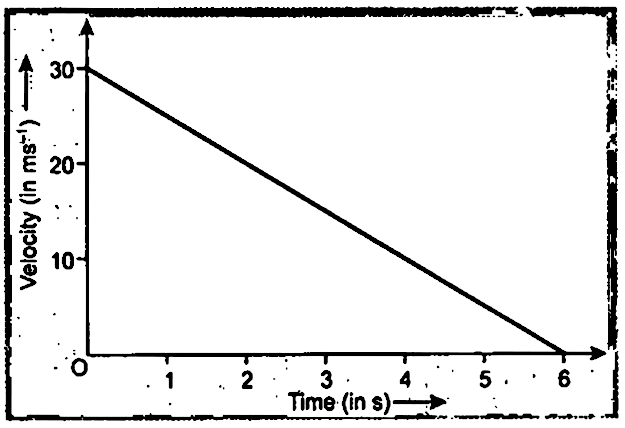Home/Class 9/Physics/

The velocity-time graph of a ball moving on the surface of a floor is shown in the figure. Find the force acting on the ball if the mass of the ball is $$50\ g$$Speed
00:00
04:06## QuestionPhysicsClass 9

The velocity-time graph of a ball moving on the surface of a floor is shown in the figure. Find the force acting on the ball if the mass of the ball is $$50\ g$$Given that,
Weight $$m=50\ g=\frac{50}{1000}=\frac{1}{20}\ kg$$
We know that slope of given $$v-t$$ graph will be acceleration.
Acceleration of the ball $$a=\frac{0-30}{6}=-5\ m\ s^{-2}$$
here $$-ve$$ sign represent that body is retarded.
$$a=5\ m\ s^{-2}$$
Force acting on the ball $$F=ma=\frac{1}{20}\times (-5)=-0.25\ N$$
Here $$-ve$$ sign indicates that the force is retarding or stopping force.